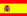You are here : > Home> Utilities > Economic and financial analysis >Investment analysis - Net Present Value method - NPV>

### Investment analysis - Net Present Value method - (NPV)

To evaluate an investment by the net present value method; enter the values in the chronological order of events, separated by commas and the Minimum Attractive Rate of Return for the periods.
Use the point as decimal separator. Ex. For the number 25,252.42, enter 25252.42;
The Minimum Attractive Rate of Return (MARR) is the lowest rate of return acceptable by investors in alternative investments or projects.
The initial value should be introduced with a negative sign.
For the periods, where no exist events, fill (0) zero.
The net present value (NPV) is a cash flow in the future, representing a value in the present, using a discount rate.
The net present value, positive, represents an investment or projects economically attractive and, if negative, indicates not economically attractive.
The results are displayed automatically, after a click on "Calculate".

 Net Present Value (NPV) Investment name: Discount rate: Elements: Total elements: Net present value (NPV):